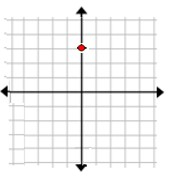# Naming the quadrant or axis of a point given its graph

If both coordinates are positive, the point must be located in quadrant 1.

If only one coordinate is positive, the point is either in quadrant 2 or quadrant 4.

If only the first coordinate is positive, then the point is in quadrant 4.

If only the second coordinate is positive, then the point is in quadrant 2.

If both coordinates are negative, the point is located in quadrant 3.

If one coordinate is zero, then the point is located on the x-axis or the y-axis.

If y coordinate is zero, then the point is located on the y-axis.

If both coordinates are zero, then the point represents the origin.

The axes, i.e., x axis and y axis divide the coordinate plane into four quadrants.

Points in the coordinate plane lie either on an axis or in one of the four quadrants.

In this lesson, we are given a grid in which a point is shown. We have to find in which quadrant the given point lies or on which axis the given point lies.

Name the quadrant or axis of the point in the following graph.### Solution

Step 1 − The point in the graph has coordinates (0, 3).

Step 2 − Since its x coordinate is zero, and y coordinate is positive, it lies on the positive y axis.

Name the quadrant or axis of the point in the following graph### Solution

Step 1 − The point in the graph has coordinates (−3, −2).

Step 2 − Since both coordinates of the point are negative, the point lies in the quadrant 3.